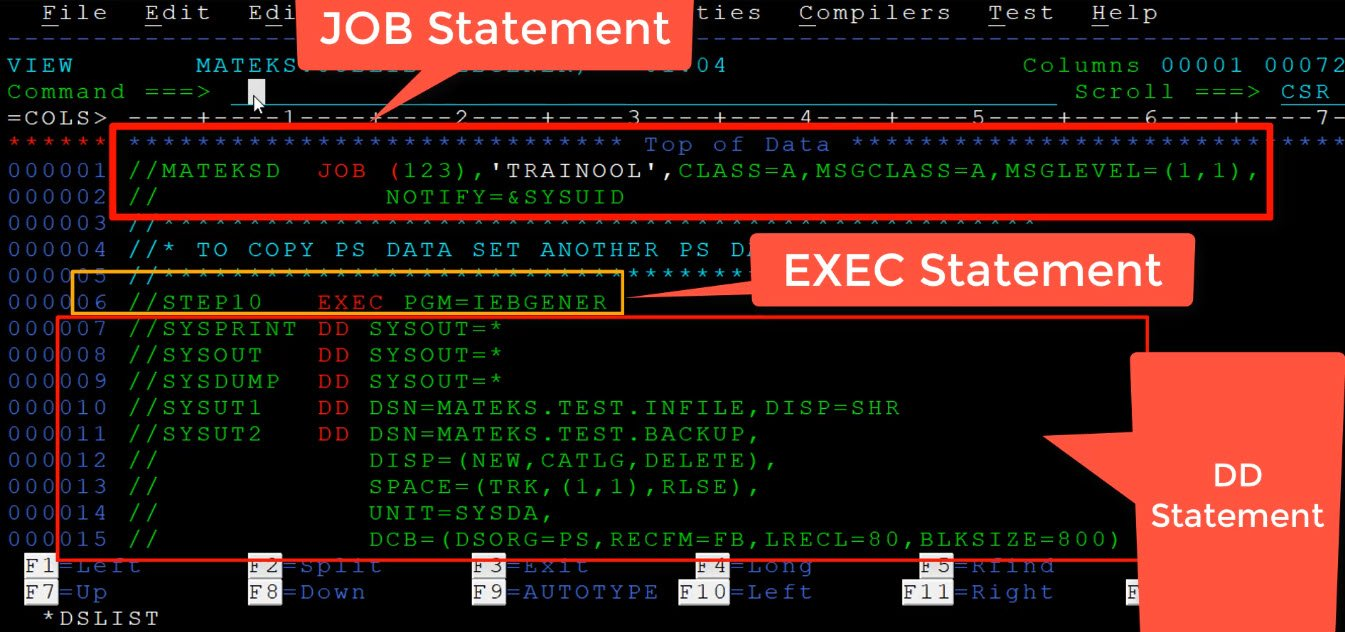# JCL Structure

## Structure of JCL• JCL statements are coded in 80-byte records. This means that you have to create a PS file or member of a PDS with a record length of 80 to code a JCL.
• Out of the 80 character records, only the first 72 characters are used to code the JCL statements.
• Every JCL starts with a Job Card.
• The JOB Card contains the  Job name which identifies the name of the job. The JOB name in most of the cases are unique but it is not necessary to give a unique name.
• And JOB card also contains the msgclass, msglevel, accounting details and other parameters which are coded based on the requirement of the JOB.
• There are 2 types of parameters used in a JCL – Positional Parameter and Keyword Parameter
• There is only one JOB card in a JCL
• A JOB card is followed by an EXEC statement which marks the beginning of a step in JCL. This step is used to perform a particular task.
• EXEC stands for Execution and there can be one or more EXEC statements in a JCL.
• Every EXEC statement contains a set of DD Statements. DD stands for Data Definition which contains the details of the datasets and the data used in the JCL.

## Example of a JOB Card

```//MATEKSD JOB (123),'TUTORIALBRAIN',CLASS=A,MSGCLASS=A,MSGLEVEL=(1,1),
// NOTIFY=&SYSUID
```

## Example of an EXEC and DD Statement in JCL

```//STEP10 EXEC PGM=IEFBR14
//SYSPRINT DD SYSOUT=*
//SYSOUT DD SYSOUT=*
//SYSDUMP DD SYSOUT=*
//DD1 DD DSN=MATEKS.TEST.PS,
//DISP=(NEW,CATLG,DELETE),
//SPACE=(TRK,(1,1),RLSE),
//UNIT=SYSDA,
//DCB=(DSORG=PS,RECFM=FB,LRECL=80,BLKSIZE=800)
```

In the Example above, there is an EXEC Statement and multiple DD statements under this EXEC Statement.

1) //STEP10 EXEC PGM=IEFBR14
This is the EXEC statment with the name of the step as ‘STEP10’. This contains a utility program ‘IEFBR14’.

2) There are four DD Statements here under the same EXEC Statement.

//SYSPRINT DD SYSOUT=*
//SYSOUT    DD SYSOUT=*
//SYSDUMP DD SYSOUT=*
//DD1 DD DSN=MATEKS.TEST.PS,
//DISP=(NEW,CATLG,DELETE),
//SPACE=(TRK,(1,1),RLSE),
//UNIT=SYSDA,
//DCB=(DSORG=PS,RECFM=FB,LRECL=80,BLKSIZE=800)
//*